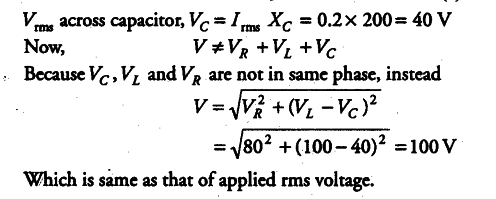# A resistor of 400 Ω

A resistor of 400 Ω, an inductor of 5/$\pi$H and a capacitor of 50/$\pi$µF are connected in series
across a source of alternating voltage of 140 sin100$\pi$tV. Find the voltage (rms) across the resistor, the inductor and the capacitor. Is the algebraic sum of these voltage more than the source voltage? If yes, resolve the paradox.

Applied voltage, V = 140 sin 100 $\pi$t V
C = 50/$\pi$µF = 50/\pi x {{10}^{-6}} F
L = 5/$\pi$H, R = 400 Ω